# rlim

Set or query r-axis limits for polar axes

## Syntax

``rlim(limits)``
``rlim('auto')``
``rlim('manual')``
``rl = rlim``
``m = rlim('mode')``
``___ = rlim(pax,___)``

## Description

example

````rlim(limits)` specifies the r-axis limits for the current polar axes. Specify `limits` as a two-element vector of the form ```[rmin rmax]```, where `rmax` is a numeric value greater than `rmin`.```

example

````rlim('auto')` lets MATLAB® choose the r-axis limits. This command sets the `RLimMode` property for the polar axes object to `'auto'`.```
````rlim('manual')` prevents the limits from changing automatically. Use this option if you want to retain the current limits when adding new data to the polar axes using the ```hold on``` command. This command sets the `RLimMode` property for the polar axes object to `'manual'`.```

example

````rl = rlim` returns a two-element vector containing the limits for the current polar axes.```
````m = rlim('mode')` returns the current value of the limits mode, which is either `'auto'` or `'manual'`. By default, the mode is automatic unless you specify limits or set the mode to manual.```

example

````___ = rlim(pax,___)` uses the polar axes specified by `pax` instead of the current polar axes. Specify `pax` as the first input argument. Include additional input or output arguments only if the original syntax supported them.```

## Examples

collapse all

Create a polar plot and change the r-axis limits.

```theta = 0:0.01:2*pi; rho = sin(2*theta).*cos(2*theta); polarplot(theta,rho) rlim([0 1])```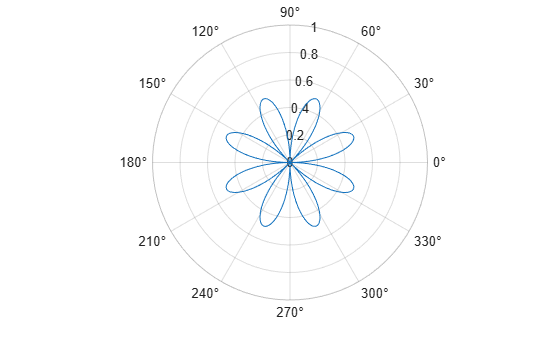Set the limits back to the original values.

`rlim('auto')`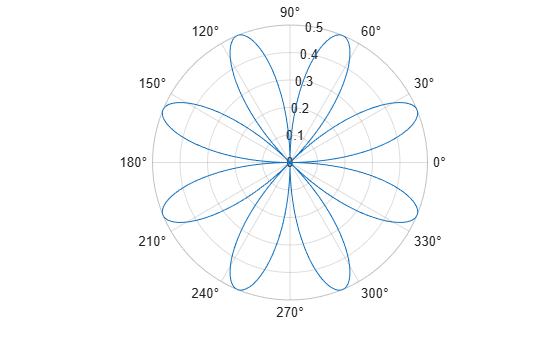Create a polar plot using negative radius values. By default, `polarplot` reflects negative values through the origin.

```theta = linspace(0,2*pi); rho = sin(theta); polarplot(theta,rho)```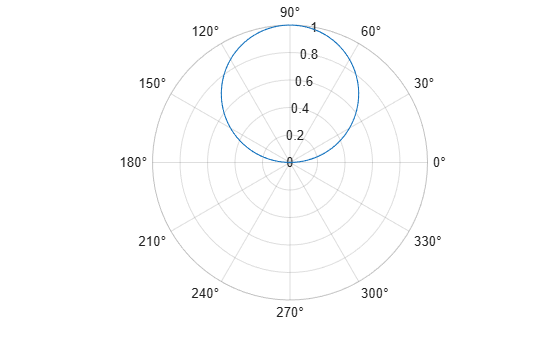Change the limits of the r-axis so it ranges from -1 to 1.

`rlim([-1 1])`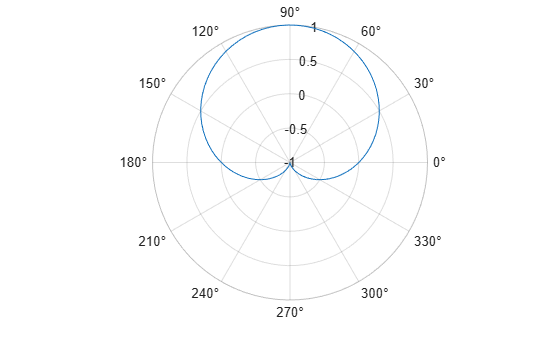Create a polar plot and return the r-axis limits.

```theta = 0:0.01:2*pi; rho = sin(2*theta).*cos(2*theta); polarplot(theta,rho)````rl = rlim`
```rl = 1×2 0 0.5000 ```

Set the limits for a specific polar axes by specifying the polar axes object as the first input to `rlim`. Otherwise, `rlim` sets the limits for the current axes.

```pax = polaraxes; rlim(pax,[0 5])```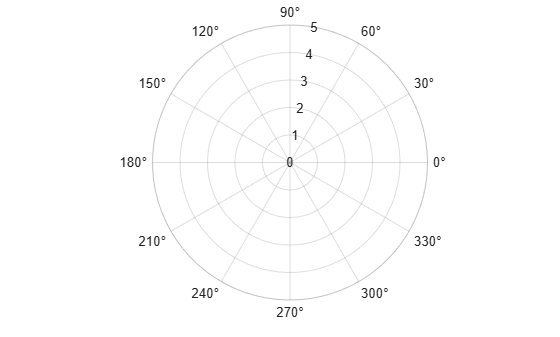## Input Arguments

collapse all

Minimum and maximum limits, specified as a two-element vector of the form `[rmin rmax]`, where `rmax` is a numeric value greater than `rmin`. You can specify both limits, or specify one limit and let MATLAB automatically calculate the other.

• To automatically set the minimum limit to the minimum data value, specify the first element as `-inf`, for example, `rlim([-inf 0])`.

• To automatically set the maximum limit to the maximum data value, specify the second element as `inf`, for example, `rlim([0 inf])`.

When you specify the limits, the `RLim` property for the polar axes object updates to the specified values and the `RLimMode` property changes to `'manual'`.

Example: `rlim([0 1])`

Polar axes, or an array of polar axes. If you do not specify this argument, then `rlim` sets the limits for the current axes (provided that the current axes is a polar axes object).

## Output Arguments

collapse all

Current limits, returned as a two-element vector of the form `[rmin rmax]`. Querying the limits returns the value of the `RLim` property for the polar axes object.

Current limits mode, returned as one of these values:

• `'auto'` — The limits automatically update to reflect changes in the data.

• `'manual'` — The limits do not automatically update to reflect changes in the data.

Querying the r-axis limits mode returns the value of the `RLimMode` property for the polar axes object.

### Properties

Introduced in R2016a